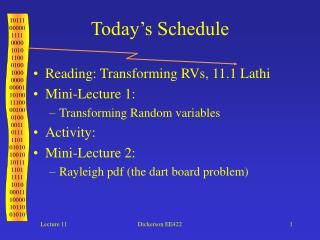# Today’s Schedule - PowerPoint PPT PresentationDownload PresentationToday’s Schedule

Download Presentation## Today’s Schedule

- - - - - - - - - - - - - - - - - - - - - - - - - - - E N D - - - - - - - - - - - - - - - - - - - - - - - - - - -
##### Presentation Transcript

1. Today’s Schedule • Reading: Transforming RVs, 11.1 Lathi • Mini-Lecture 1: • Transforming Random variables • Activity: • Mini-Lecture 2: • Rayleigh pdf (the dart board problem) Dickerson EE422

2. Functional Transformations of RVs • RV’s need to be evaluated as a function of another RV whose distribution is known h(x) Transfer Characteristic (no memory) x Input PDF, fx(x), given y=h(x) Output PDF, fy(y), to be found Dickerson EE422

3. Transforming RVs • Theorem: If y=h(x) where h( ) is the transfer function of a memoryless device, Then the PDF of the output, y is: • M is the number of real roots of y=h(x), which means that y=h(x) gives x1, x2,. . ., xMfor a single value of y. Dickerson EE422

4. Example Sinusoidal Distribution • Let • x is uniformly distributed from –pi to pi Dickerson EE422

5. Analysis • For some value of y, say y0, there are two values of x, say x1and x2 Dickerson EE422

6. Output PDF • Simplify by replacing pdf of x with 1/2p • Evaluating cosine terms, see figure Dickerson EE422

7. Dickerson EE422

8. Diode current-voltage characteristic modeled as shown B>0 For y>0, M=1; y<0 M=0 At y=0, it maps to all x<0 Diode Characteristic Dickerson EE422

9. Finding the PDF Dickerson EE422

10. Activity1 • y=Kx • X is normal, N(0,sx2) • Find the pdf of y Dickerson EE422

11. Dart Board • Randomly throw darts at a dart board • More likely to throw darts in center each coordinate is a Gaussian RV Dickerson EE422

12. To put it another way . . . • Given two independent, identically distributed (IID) Gaussian RVs, x and y: • Find the PDFs of the amplitude and phase of these variables (polar coordinates): Dickerson EE422

13. Example Rayleigh Distribution • Joint density of x and y is: • Transform from (x,y) to polar coordinates: Dickerson EE422

14. Probability of hitting a spot C y dq q dr dq dr x r Dickerson EE422

15. Change Coordinates • From calculus recall that this integral can be converted to polar coordinates: Dickerson EE422

16. Change Coordinates • Relationship between density functions is: Dickerson EE422

17. Change Coordinates • Relationship between density functions is: Dickerson EE422

18. Marginal Densities Take the joint distribution and integrate out one of the variables: Dickerson EE422

19. Rayleigh Distribution • Rayleigh distribution; used to model fading, radar clutter Dickerson EE422

20. Activity 2 • y=x2 • Find the pdf of y • X is normal, N(0,sx2) Dickerson EE422

21. Next Time • Reading: Lathi 11.1,11.2 • Mini-Lecture 1: • Random processes • Activity: • Mini-Lecture 2: • Power spectral density of random processes Dickerson EE422